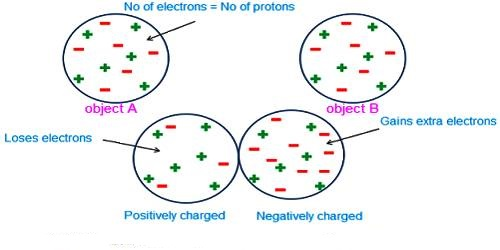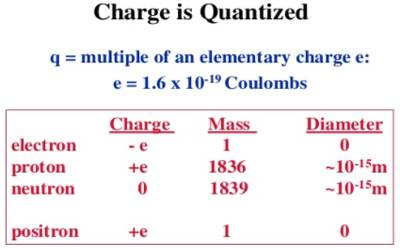# Quantization of Charge

Quantization of charge

Quantization of charge means that when we say something has a given charge, we mean that that is how many times the charge of a single electron it has. The charge of an electron or a proton is the minimum charge available in nature. The charge of an electron is designated as (- e) and the charge of a proton is designated as (+ e). Its magnitude is e = 1.60218 x 10-19 C.It is seen by experiment that total charge in a body in nature is an integral multiple of a minimum amount of charge. This minimum amount is the charge of an electron. All charges that exist in all charged bodies are the integral multiple of this smallest charge, i.e., the multiple of the charge of an electron. It is called the quantization of charges. Any amount of charge cannot exist in a body. If e is the charge of an electron, then total charge in a body, q = ne; where n is a positive or negative integer. For example, the 1-coulomb charge is equal to the charge of 6.24 x 1018 electrons. In nature, there is no existence of a fraction of the value of e. For example, 2.5e, — 3.7e etc. amount of charge is not available in nature.

For example, in a hydrogen ion, we usually denote it with a positive sign to indicate that there’s one proton more than there are electrons.